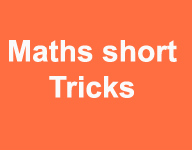# How to solve the cube root with short trickHow to solve the cube root with short trick

Now we are display the short trick of cube root. The cube root find type question in exam very time consuming. Cube root related question put in maximum computational exams .If we solve the cube root question with proper basic steps .so more time waist in exam and other question cannot attempt .So number of trick are available to solve the math’s questions and now we are discussed the how to solve the cube root with short trick method .

So first write the cube root for 1 to 10

Daily Visit GK on myshort.in

1= 1

2= 8

3= 27

4 = 64

5 = 125

6 = 216

7 = 343

8 = 572

9 = 729

10 = 1000

Question 1.Step 1 .Select the last 3 digit and then check the last digit and find the cube root for last digit match

In question last number 648 = and last number 8

So=  8

Step 2. Now select the first 2 digit 10

So find the cub root of nearest digit of 10 that is 8 because cube root of 10 is not available.

So cube root 2 =  8 , so select 2

Ans    22

Question 2.Step 1. Select last three digit 039 and cube root is last number 9 is available in 9 cube root=739

039 =  9

Step 2. Select the first three digits

493 = value is nearest by cube root by 7 = 343

Ans 79

Note we are not accept the cube root of 8 = 572 because it is greater then actule value of 493 so we are accept the lowest value not greatest value .

Question 3Select last 3 digit 640 = in case if last number 0 so find the approximate value of 0

Nearest by 1 ,9, 5

Select first 2 digit 10 = nearest cube of 8 = 2

So select the ans in 21,29,25

Ans 21  nearest

Question 4=

357 = last number in cube root= 3 = 27

804 = number is nearest by cube root of 9 = 729

Ans  = 93

Buy online Rajasthan gk book Railway JE CBT REET PAtwari Book SSC CGL Clerk GD Book Buy online Rajasthan gk book Railway JE CBT REET PAtwari Book SSC CGL Clerk GD Book